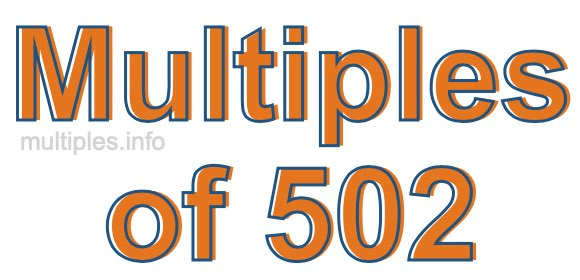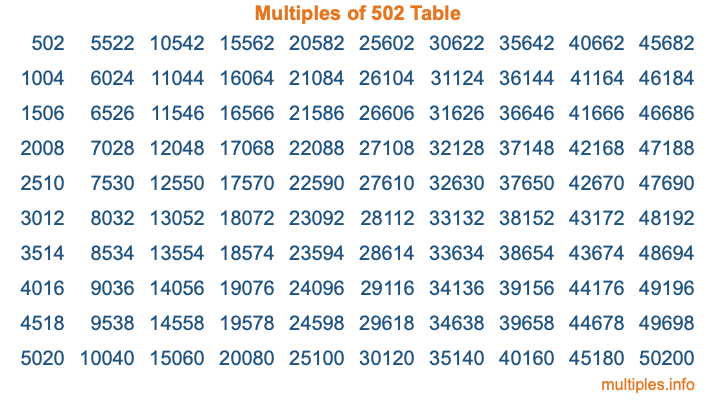Multiples of 502Welcome to the Multiples of 502 page. Here we will first teach you everything you will ever need to know about the multiples of 502, and then give you a study guide summary of everything we taught you to make sure you remember it all. Use this page to look up facts and learn information about the multiples of 502. This page will make you a multiples of five hundred two expert!

Definition of Multiples of 502
Multiples of 502 are all the numbers that when divided by 502 equal an integer. Each of the multiples of 502 are called a multiple. A multiple of 502 is created by multiplying 502 by an integer.

Therefore, to create a list of multiples of 502, you start with 1 multiplied by 502, then 2 multiplied by 502, then 3 multiplied by 502, and so on for as long as you want. Thus, the list of the first five multiples of 502 is 502, 1004, 1506, 2008, and 2510. To see a larger list of multiples of 502, see the printable image of Multiples of 502 further down on this page. We also have a category where you can choose any nth multiple of 502.

Multiples of 502 Checker
The Multiples of 502 Checker below checks to see if any number of your choice is a multiple of 502. In other words, it checks to see if there is any number (integer) that when multiplied by 502 will equal your number. To do that, we divide your number by 502. If the the quotient is an integer, then your number is a multiple of 502.

Is  a multiple of 502?

Least Common Multiple of 502 and ...
A Least Common Multiple (LCM) is the lowest multiple that two or more numbers have in common. This is also called the smallest common multiple or lowest common multiple and is useful to know when you are adding our subtracting fractions. Enter one or more numbers below (502 is already entered) to find the LCM.

Check out our LCM Calculator if you need more details about the Least Common Multiple or if you need the LCM for different numbers for adding and subtraction fractions.

nth Multiple of 502
As we stated above, 502 is the first multiple of 502, 1004 is the second multiple of 502, 1506 is the third multiple of 502, and so on. Enter a number below to find the nth multiple of 502.

th multiple of 502

Multiples of 502 vs Factors of 502
502 is a multiple of 502 and a factor of 502, but that is where the similarities end. All postive multiples of 502 are 502 or greater than 502. All positive factors of 502 are 502 or less than 502.

Below is the beginning list of multiples of 502 and the factors of 502 so you can compare:

Multiples of 502: 502, 1004, 1506, 2008, 2510, etc.

Factors of 502: 1, 2, 251, 502

As you can see, the multiples of 502 are all the numbers that you can divide by 502 to get a whole number. The factors of 502, on the other hand, are all the whole numbers that you can multiply by another whole number to get 502.

It's also interesting to note that if a number (x) is a factor of 502, then 502 will also be a multiple of that number (x).

Multiples of 502 vs Divisors of 502
The divisors of 502 are all the integers that 502 can be divided by evenly. Below is a list of the divisors of 502.

Divisors of 502: 1, 2, 251, 502

The interesting thing to note here is that if you take any multiple of 502 and divide it by a divisor of 502, you will see that the quotient is an integer.

Multiples of 502 Table
Below is an image of the first 100 multiples of 502 in a table. The table is in chronological order, column by column. The first column has the first ten multiples of 502, the second column has the next ten multiples of 502, and so on.The Multiples of 502 Table is also referred to as the 502 Times Table or Times Table of 502. You are welcome to print out our table for your studies.

Negative Multiples of 502
Although not often discussed or needed in math, it is worth mentioning that you can make a list of negative multiples of 502 by multiplying 502 by -1, then by -2, then by -3, and so on, to get the following list of negative multiples of 502:

-502, -1004, -1506, -2008, -2510, etc.

Multiples of 502 Summary
Below is a summary of important Multiples of 502 facts that we have discussed on this page. To retain the knowledge on this page, we recommend that you read through the summary and explain to yourself or a study partner why they hold true.

There are an infinite number of multiples of 502.

A multiple of 502 divided by 502 will equal a whole number.

502 divided by a factor of 502 equals a divisor of 502.

The nth multiple of 502 is n times 502.

The largest factor of 502 is equal to the first positive multiple of 502.

502 is a multiple of every factor of 502.

502 is a multiple of 502.

A multiple of 502 divided by a divisor of 502 equals an integer.

502 divided by a divisor of 502 equals a factor of 502.

Any integer times 502 will equal a multiple of 502.

Multiples of a Number
Here you can get the multiples of another number, all with the same attention to detail as we did for multiples of 502 on this page.

Multiples of
Multiples of 503
Did you find our page about multiples of five hundred two educational? Do you want more knowledge? Check out the multiples of the next number on our list!